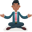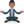Trusted answers to developer questions
Trusted Answers to Developer Questions

Related Tags

python

# What is numpy.bincount() in Python?Abhilash

Grokking Modern System Design Interview for Engineers & Managers

Ace your System Design Interview and take your career to the next level. Learn to handle the design of applications like Netflix, Quora, Facebook, Uber, and many more in a 45-min interview. Learn the RESHADED framework for architecting web-scale applications by determining requirements, constraints, and assumptions before diving into a step-by-step design process.

The bincount() method of the NumPy module is used to find the frequency of each element in a NumPy array of positive integers. The element’s index in the frequency array or bin is stored as the element’s count. Because each bin value represents an instance of its index, we can adjust the bin size accordingly. This method is beneficial for counting vast amounts of data or records.

The length of the resulting array will always be  1 + maximum element in the array.

The method throws an error if the input array has negative or floating point elements.

### Syntax

numpy.bincount(x, weights=None, minlength=0)


### Parameters

• x: This is a positive dimension array.
• weights: The weights array has to be the same dimension as x.
• minlength: This is the minimum number of bins for the output array.

### Return value

This method returns the frequency array or bin.

### Code

import numpy as nparr = np.array([4, 4, 2, 1, 6, 8, 3, 3, 4, 15, 23])freq_bin = np.bincount(arr)print("Input array - ", arr)print("Frequency bin/array - ", freq_bin)print("Length of Frequency bin/array - ", len(freq_bin))

### Explanation

• Line 1: Import the numpy module.
• Line 2: The input array of positive integers is defined.
• Line 3: The frequency bin/array is calculated using the numpy.bin() method.
• Line 4: The input array is printed.
• Line 5: The frequency bin/array is printed.
• Line 6: The frequency bin/array length is printed. The length of the frequency bin is 1 + 23 (maximum element in the array).

RELATED TAGS

python

CONTRIBUTORAbhilash